# What is the pixel LED bulb.

Hello guys, welcome back to my SriTu Hobby blog. Today we will be going to learn what is pixel LED bulbs. These pixel LED bulbs are different from ordinary LED bulbs. Because we can get any color from these pixel LEDs.This pixel LED bulb includes three main colors. That is (RGB) Red, Green, and Blue. We can get any color by increasing and decreasing these main colors. But we can’t buy these pixel LEDs one by one. These are available in strips and string. But we can access the LED bulbs in these strips and strings one by one. Because of this reason, these are called addressable LEDs. That is, we can control the LED bulbs one by one. We can do this because of the IC in the bulbs. These ICs are mainly two types in the market. That is WS2811 and WS2812. We can control these pixel LEDs using three wires. So, two wires are used to supply power and the other wire is used to provide the signals.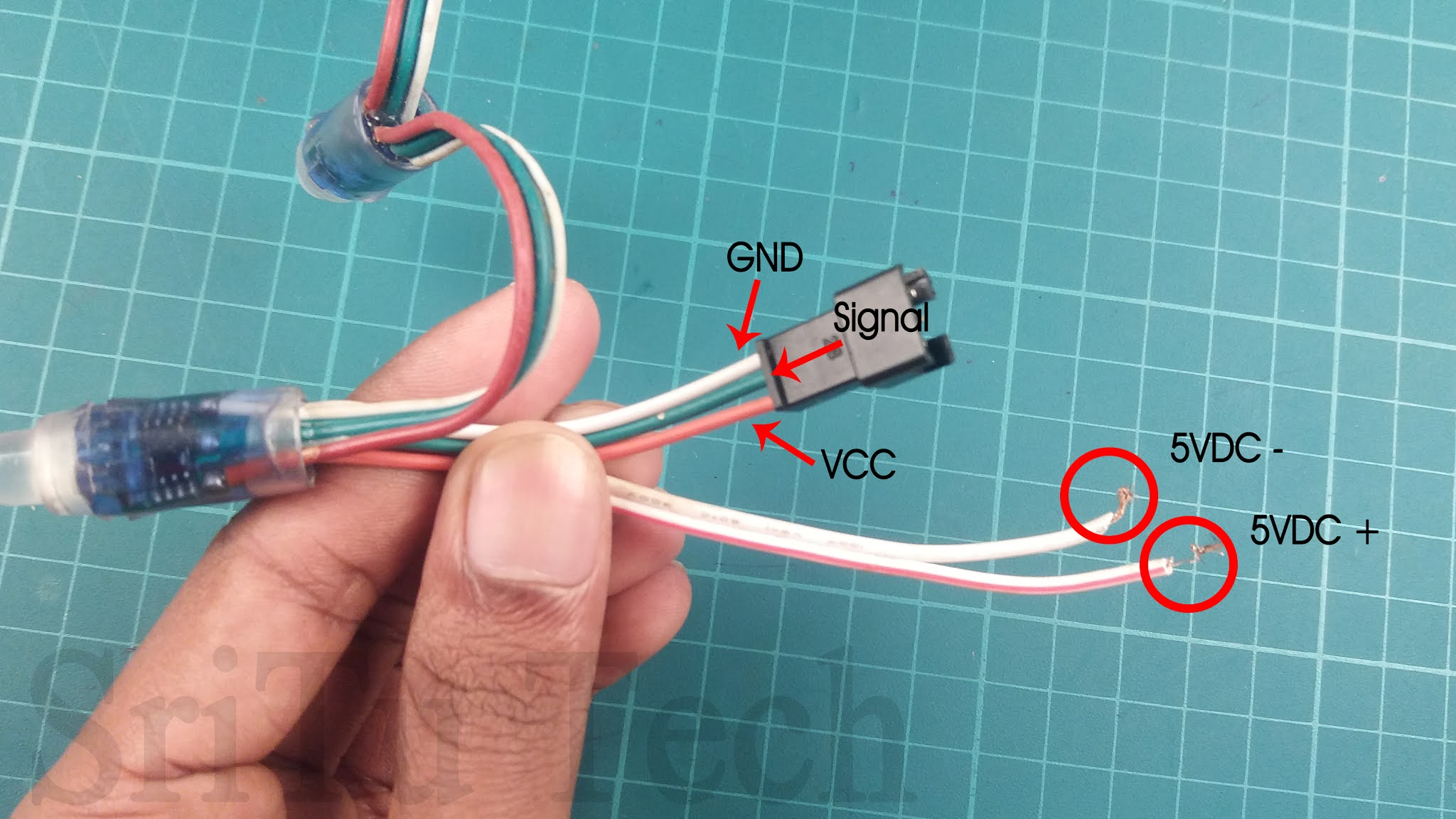One more thing, there are two types of power to be given to these pixel LEDs. That is 5v and 12v. Remember to apply the correct voltage, or it will burn out. Today we are going to use a pixel LED string that includes the WS2811 IC. For that, we need to provide a 5v DC voltage. From this string, we can see 50 LED bulbs and a connector at the end. Through that, we can connect pixel LED strings continuously.Especially, these LED bulbs do not work from normal power. For that, we need to provide signals. We can buy controlling boards made for this purpose in the market. But today we will use the Arduino Nano board for that. It is very low cost and easy to use. So, let’s do this project. The required components are as follows. You can easily buy these components using the links below.

### Components

Let’s learn this lesson step by step.

### Step 1

Let’s identify these components.
Arduino Uno board.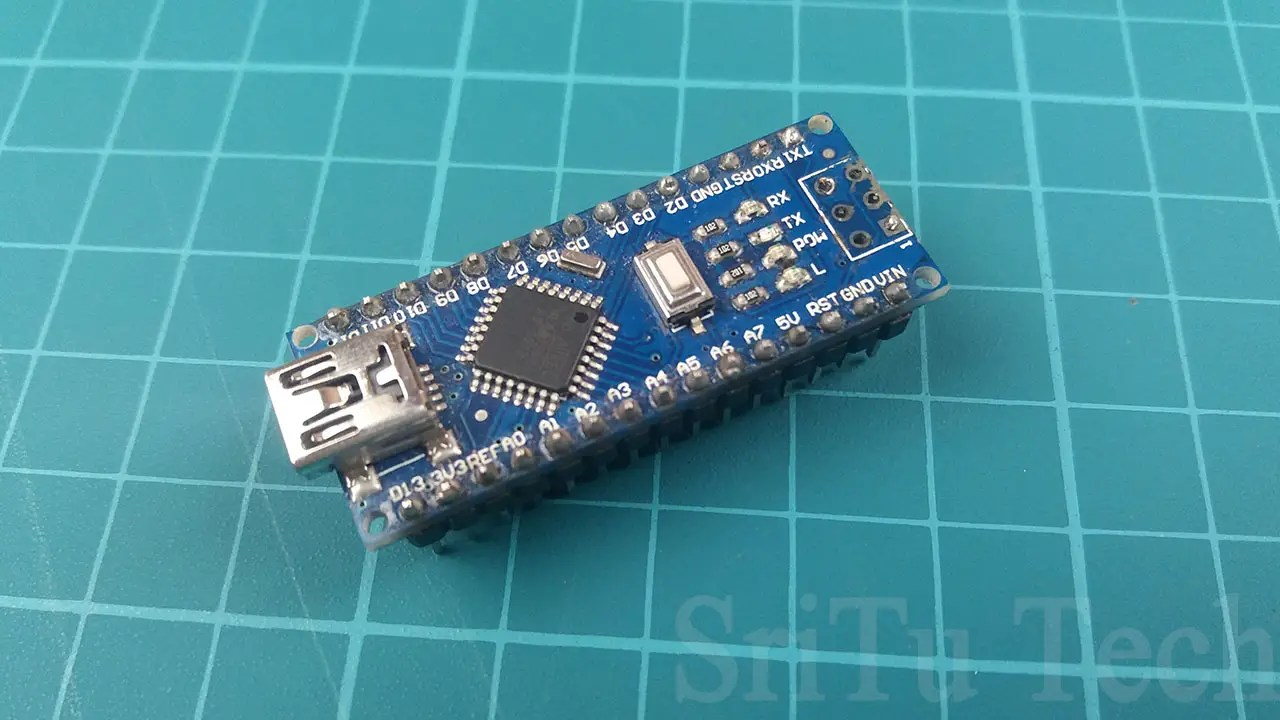You can use any other Arduino board.
Pixel LED string.This component is described above.
Jumper wires.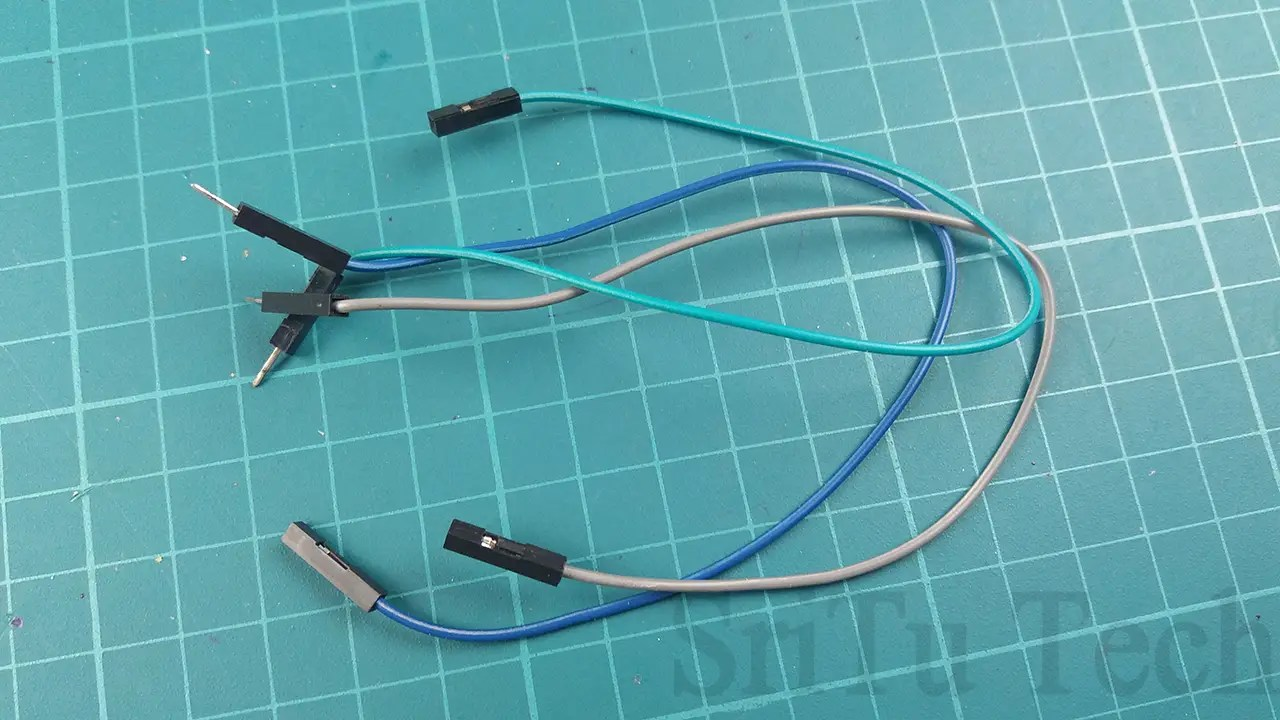For that, I use three male to female jumper wires.

### Step 2

Connect this pixel LED string to the Arduino Nano board. For that, using the circuit diagram below. But you can use any digital PIN for this project.

### Step 3

Now connect it to the computer using a USB cable. Then, download the “Fast LED” library and insert it into the Arduino IDE. Download this library using this link. Okay, let’s look at the code below.
The complete program of this project – Download
``````/*pixel LED control with Arduino nano.
created by the SriTu Tech team.
Read the code below and use it for any of your creation
*/
#include <FastLED.h>//include library---library link in my blog
#define LED 50//num of leds
#define DATAPIN 3//define arduino pin
#define chip WS2811 //chip name
#define colorArrange RGB//color
CRGB pixel [LED];//create array
void setup() {
FastLED.addLeds<chip, DATAPIN, colorArrange>(pixel, LED);//initializing pixel led
}

void loop() {
runRed();
runGreen();
runBlue();
Fill();
}
void runRed() {
for (byte a = 0; a <= 50; a ++ ) {
pixel[a] = CRGB::Red;
pixel[a + 1] = CRGB::Red;
FastLED.show();
delay(50);
pixel[a] = CRGB::Black;
pixel[a + 1] = CRGB::Black;
}
}
void runGreen() {
for (byte x = 0; x <= 50; x ++ ) {
pixel[x] = CRGB::Green;
pixel[x + 1] = CRGB::Green;
FastLED.show();
delay(50);
pixel[x] = CRGB::Black;
pixel[x + 1] = CRGB::Black;
}
}
void runBlue() {
for (byte x = 0; x <= 50; x ++ ) {
pixel[x] = CRGB::Blue;
pixel[x + 1] = CRGB::Blue;
FastLED.show();
delay(50);
pixel[x] = CRGB::Black;
pixel[x + 1] = CRGB::Black;
}
}
void Fill() {
for (byte a = 0; a <= 50; a++) {
pixel[a] = CRGB::Red;
FastLED.show();
delay(30);
}
for (byte b = 0; b <= 50; b++) {
pixel[b] = CRGB::Green;
FastLED.show();
delay(30);
}
for (byte c = 0; c <= 50; c++) {
pixel[c] = CRGB::Blue;
FastLED.show();
delay(30);
}
for (byte d = 0; d <= 50; d++) {
pixel[d] = CRGB::Yellow;
FastLED.show();
delay(30);
}
}
for (int w = 0; w <= 50; w++) {
pixel[w] = CRGB(0, 0, 255);
pixel[w + 1] = CRGB(0, 0, 230);
pixel[w + 2] = CRGB(0, 0, 210);
pixel[w + 3] = CRGB(0, 0, 190);
pixel[w + 4] = CRGB(0, 0, 150);
FastLED.show();
delay(50);
pixel[w] = CRGB(0, 0, 0);
pixel[w + 1] = CRGB(0, 0, 0);
pixel[w + 2] = CRGB(0, 0, 0);
pixel[w + 3] = CRGB(0, 0, 0);
pixel[w + 4] = CRGB(0, 0, 0);
FastLED.show();
}
}``````
This code the first time includes the pixel LED controlling library. Next, we define how many LED bulbs to use. After, define the data pin, chip name and color arrange. The code in the void setup function initiates the pixel LED string. Read and understand other code lines. They are included in the “Fast LED” library file.

### Step 4

Next, select the correct board and port.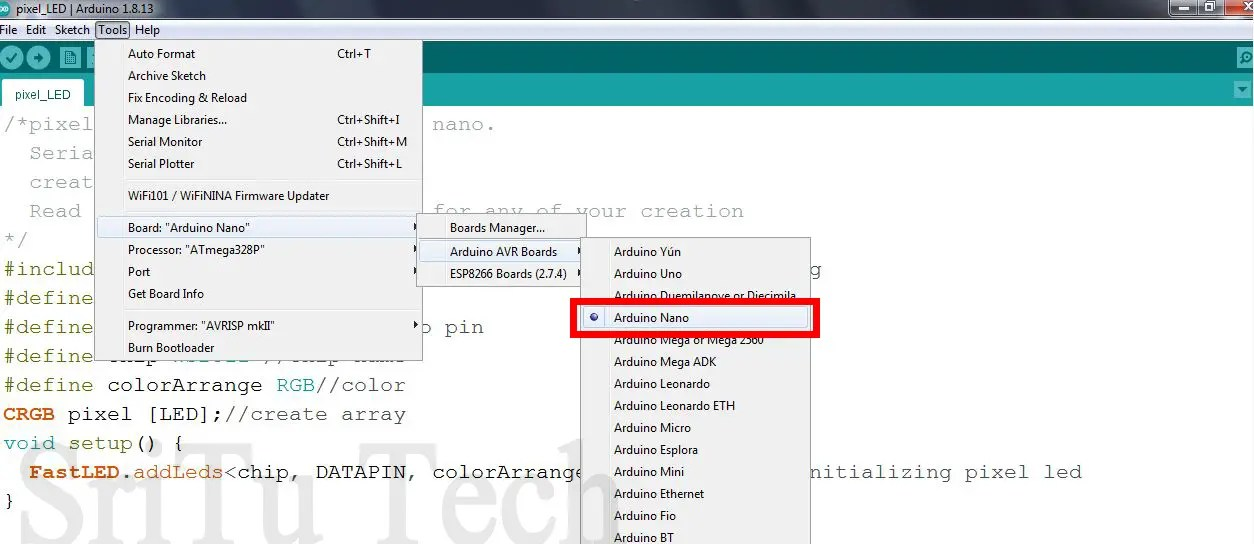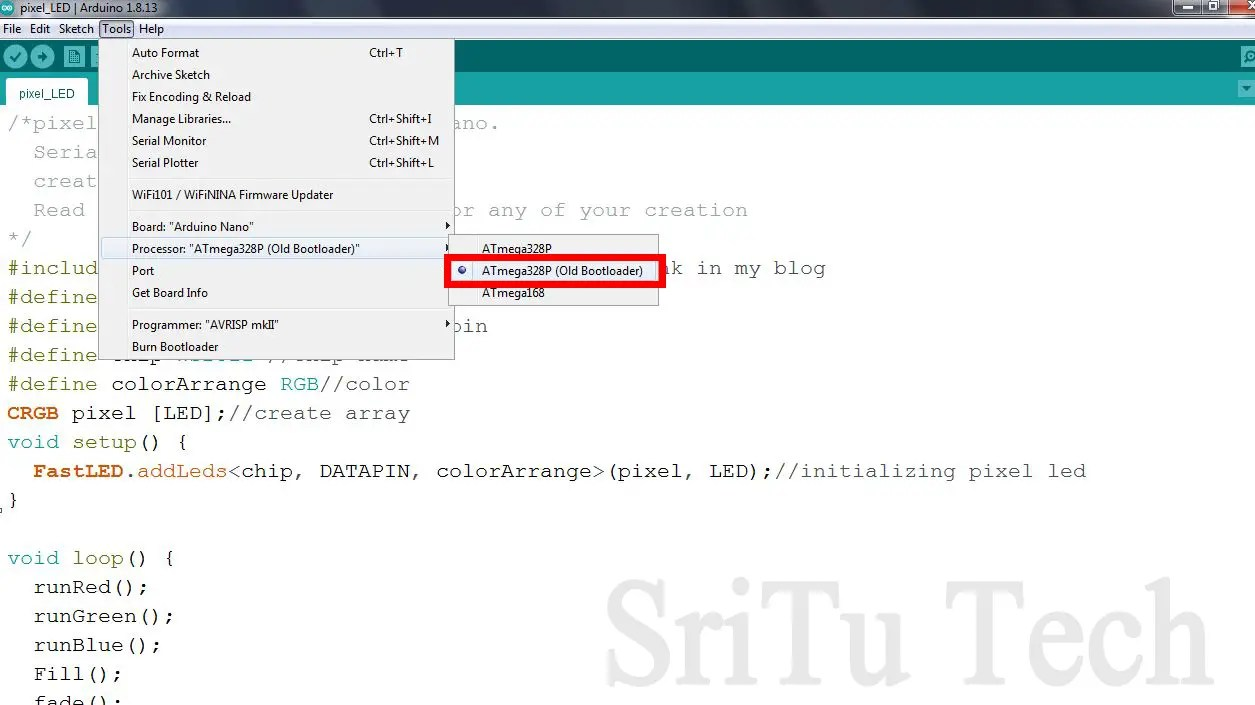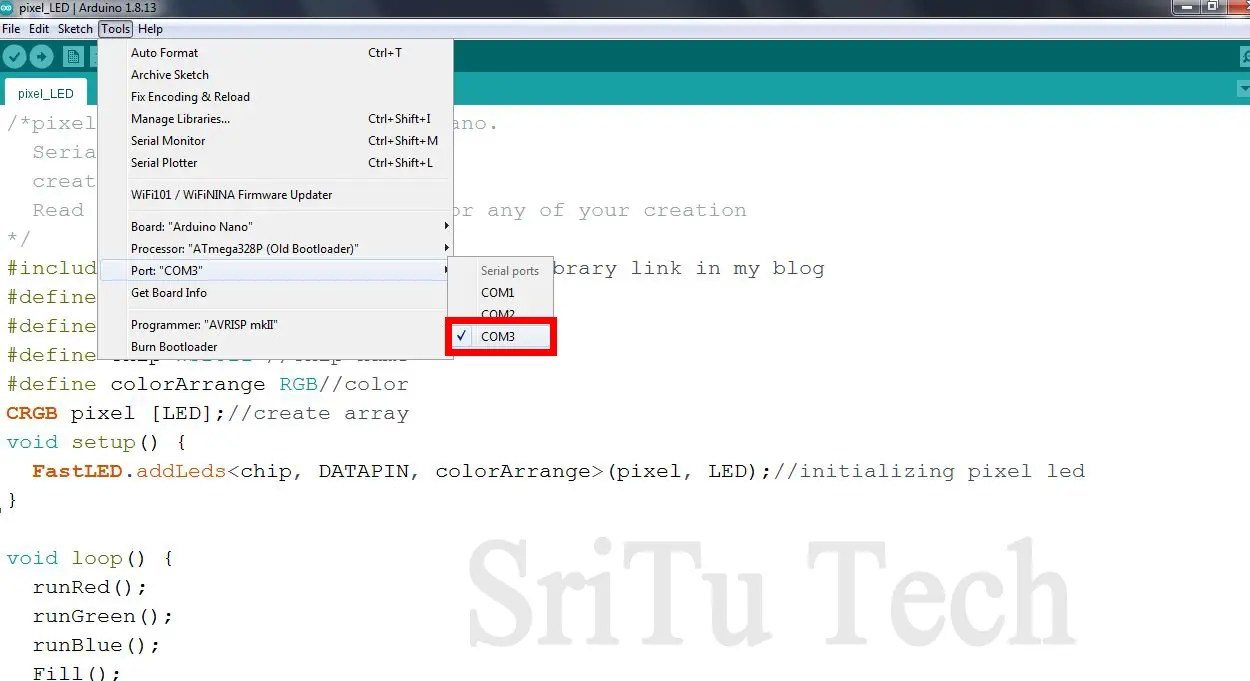### Step 5

Finally, upload this code.Watch the full video guide below. We will meet in the next post. Have a good day. Bye-bye.

What is the pixel LED bulb.